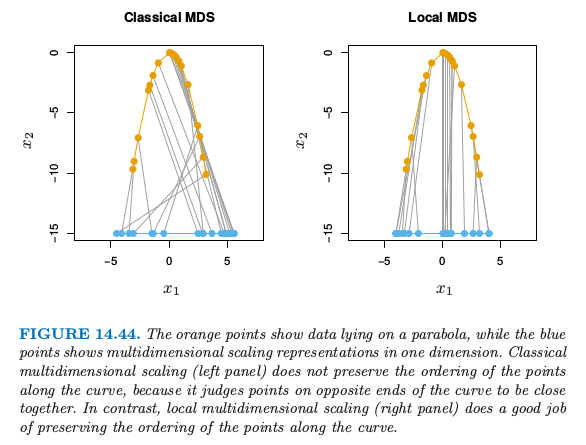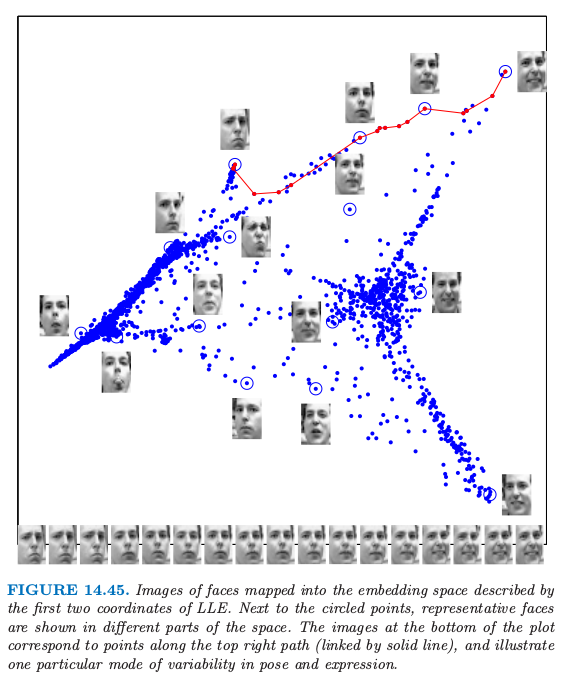# 14.9 非线性降维和局部多维缩放¶1. 对每个 $p$ 维中的数据点 $x_i$，寻找欧式距离意义下的 $K$ 最近邻点 $\cal N(i)$.
2. 对每个点用邻居点的混合仿射来近似 其中权重 $w_{ik}$ 满足 $w_{ik}=0, k\not\in \cal N(i), \sum_{k=1}^Nw_{ik}=1$．$w_{ik}$ 是点 $k$ 对 $i$ 点的重构的贡献．注意到为了得到唯一解，我们必须要求 $K < p$．
3. 最后，固定 $w_{ik}$，在 $d < p$ 维空间中寻找点 $y_i$ 来最小化

weiya 注:

weiya 注

14.7节中的谱聚类的 mutual K-nearest-neighbor graph 也有用到 $\cal N$．Sam Roweis 和 Lawrence Saul 友好地提供了图 14.45.

1. Tenenbaum, J. B., de Silva, V. and Langford, J. C. (2000). A global geometric framework for nonlinear dimensionality reduction, Science 290: 2319–2323.

2. Roweis, S. T. and Saul, L. K. (2000). Locally linear embedding, Science 290: 2323–2326.

3. Chen, L. and Buja, A. (2008). Local multidimensional scaling for nonlinear dimension reduction, graph drawing and proximity analysis, Journal of the American Statistical Association.